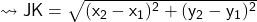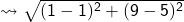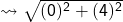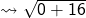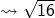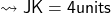## Triangle JKL is fromed by plotting three points. What is the leanth of sides JK? j(1 , 5) k(1, 9) answers > 6

Question

Triangle JKL is fromed by plotting three points. What is the leanth of sides JK?
j(1 , 5) k(1, 9)
answers > 6 3 5 2​

in progress 0
5 months 2021-08-27T07:01:08+00:00 1 Answers 6 views 0

1. ### Solution:–

• J (1,5)
• K (1,9)